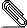# Re: [HACKERS] CUBE seems a bit confused about ORDER BY

```On Sun, Oct 29, 2017 at 1:30 AM, Alexander Korotkov <
a.korot...@postgrespro.ru> wrote:```
```
> On Fri, Oct 20, 2017 at 1:01 AM, Alexander Korotkov <
> a.korot...@postgrespro.ru> wrote:
> I've reviewed code of ~> operator and its KNN-GiST support.
> Unfortunately, it appears that it's broken in design...  The explanation is
> above.
>
> We've following implementation of ~> operator.
>
> if (coord <= DIM(cube))
>> {
>> if (IS_POINT(cube))
>> PG_RETURN_FLOAT8(cube->x[coord - 1]);
>> else
>> PG_RETURN_FLOAT8(Min(cube->x[coord - 1],
>> cube->x[coord - 1 + DIM(cube)]));
>> }
>> else
>> {
>> if (IS_POINT(cube))
>> PG_RETURN_FLOAT8(cube->x[(coord - 1) % DIM(cube)]);
>> else
>> PG_RETURN_FLOAT8(Max(cube->x[coord - 1],
>> cube->x[coord - 1 - DIM(cube)]));
>> }
>
>
> Thus, for cube of N dimensions [1; N - 1] are lower bounds and [N; 2*N -
> 1] are upper bounds.  However, we might have indexed cubes of different
> dimensions.  For example, for cube of 2 dimensions "cube ~> 3" selects
> upper bound of 1st dimension.  For cube of 3 dimensions "cube ~> 3" selects
> lower bound of 3rd dimension.
>
> Therefore, despite ~> operator was specially invented to be supported by
> KNN-GIST, it can't serve this way.
>
> Regarding particular case discovered by Tomas, I think the error is in the
> GiST supporting code.
>
> if (strategy == CubeKNNDistanceCoord)
>> {
>> int coord = PG_GETARG_INT32(1);
>> if (DIM(cube) == 0)
>> retval = 0.0;
>> else if (IS_POINT(cube))
>> retval = cube->x[(coord - 1) % DIM(cube)];
>> else
>> retval = Min(cube->x[(coord - 1) % DIM(cube)],
>> cube->x[(coord - 1) % DIM(cube) + DIM(cube)]);
>> }
>
>
> g_cube_distance() always returns lower bound of cube.  It should return
> upper bound for coord > DIM(cube).
>
> It would be also nice to provide descending ordering using KNN-GiST.  It
> would be especially effective for upper bound.  Since, KNN-GiST doesn't
> support explicit descending ordering, it might be useful to make ~>
> operator return negative of coordinate when negative argument is provided.
> For instance, '(1,2), (3,4)'::cube ~> -1 return -1.
>
> I'd like to propose following way to resolve design issue.  cube ~> (2*N -
> 1) should return lower bound of Nth coordinate of the cube while cube ~>
> 2*N should return upper bound of Nth coordinate.  Then it would be
> independent on number of coordinates in particular cube.  For sure, it
> would be user-visible incompatible change.  But I think there is not so
> many users of this operator yet.  Also, current behavior of ~> seems quite
> useless.
>

Thus, I heard no objection to my approach.  Attached patch changes ~>
operator in the proposed way and fixes KNN-GiST search for that.  I'm going
to register this patch to the nearest commitfest.

------
Alexander Korotkov
Postgres Professional: http://www.postgrespro.com
The Russian Postgres Company
```cube-knn-fix-1.patch
Description: Binary data

```--
Sent via pgsql-hackers mailing list (pgsql-hackers@postgresql.org)
To make changes to your subscription:
http://www.postgresql.org/mailpref/pgsql-hackers
```